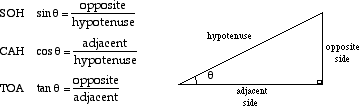# Slope Profiles Part 1: Calculating with Slope Angles

How to create a slope profile by using slope angle to calculate elevation and horizontal distance.

### Data needed ↑

A slope profile is a graph that shows changes in elevation of a slope along a vertical plane – a side view (profile) of a slope. The basic idea is this: if you knew the (1) elevation of different points of the slope, and knew the (2) horizontal distances between these points, you would be able to recreate the slope into a graph.There are several ways to collect these two sets of data. However, in practice, it is usually difficult to directly measure the elevation and horizontal distances (unless you slice your slope down its side and have a very long measuring tape, which aren’t real options). This is where slope angles come in.

### The math ↑

Remember SOH-CAH-TOA from high school math? We will use these trigonometry rules to calculate the data we need.Imagine the elevation of a certain point on the slope being the height of the “opposite side” of a right angled triangle. The length of the “adjacent side” would then be the horizontal distance, and the hypotenuse is equivalent to the slope distance.The slope distance from Point A to Point B is straightforward to measure with a tape measure. Slope angles are also easily measured using equipment such as a clinometers (or inclinometers). You can even make your own make-shift clinometer with a protractor. Total stations can measure both slope distance and slope angles (more details on measuring slope angles here).

For now, assume that you have measured slope distance and slope angle – you can now calculate elevation (using the sine rule) and horizontal distance (using the cosine or tangent rule)Tip: If using a scientific calculator, remember to calculate in degrees, not radians.

You would then have to repeat these steps (from Point B to Point C, Point C to Point D etc.) to get your full slope profile. However, remember that the elevation calculated of the second point is relative to the first point. For instance, the calculated elevation for Point C would be its height from Point B, not Point A. For your final slope profile graph, you would therefore have to add Point A’s elevation too to get your final elevation for Point C.Point D would be E1 + E2 + E3, Point E would be E1 + E2 + E3 + E4 etc.

Tip: Have enough points to show changes in slope shape (e.g. concavity, convexity, plateauing).

You may then present your data as follows, and then graph it on Microsoft Excel. Ensure that you use a Scatter graph, not a Line graph (graph both to see why!).### Common mistakes ↑

• Using a Line graph instead of a Scatter graph on Microsoft Excel.
• General graphing mistakes e.g. not labelling the axes, no units to the labels.

Slope Profiles Part 2: Measuring slope angles